# 定义

“维护一个有序数列，有插入、删除、查询第k大、查询前驱、后继等操作”，对于这种问题，我们常用到二叉排序树（BST，Binary Sort Tree，也称二叉查找树、二叉搜索树）这种数据结构，而Treap就是对它的一种优化

# 构造方法

## 思路

1. 插入一个数$k$

首先考虑满足二叉排序树的性质，从根节点开始向下查找，每找到一个节点$o$，有如下四种情况：

(1)$o$为空，即创建新节点，初始化，赋$key$$pri$值，返回；

(2)$k=o.key$$o.val+1$即可；

(3)$k<o.key$，进入该节点左儿子查找；

(4)$k>o.key$，进入该节点右儿子查找。

创建完新节点，满足了BST的性质，接下来考虑满足堆的性质，介绍如下两种旋转操作：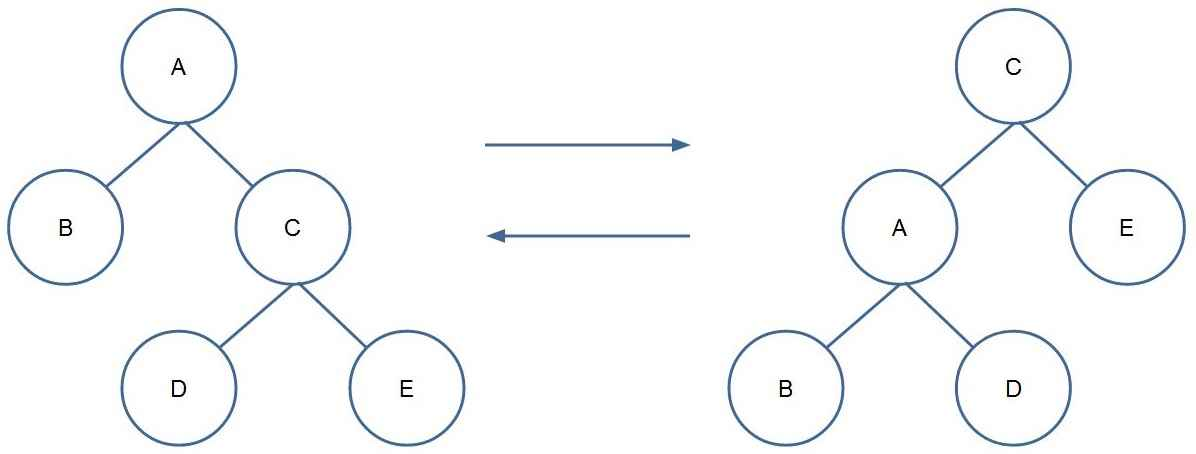上图可视为一棵二叉树的一部分，从左图到右图为左旋，反过来为右旋。

可以看出，旋转前后，这一部分的中序遍历均为$BADCE$，即旋转不破坏二叉排序树的性质，于是我们可以利用旋转操作，在保持二叉排序树的前提下，维护堆的性质。

再具体来讲，这一部分最核心的两个节点是$A$$C$，旋转时，只需要调整节点$D$的位置，再调整$A$$C$的父子关系，最后维护一下$val$$siz$值即可。

在创建完新节点之后，可以得到根节点到这个新节点的一条路径，递归回溯时，对这条路径上的每两个点：若某个左子节点($A$)的优先级比其父节点($C$)小，则进行一次右旋；若某个右子节点($C$)的优先级比其父节点($A$)小，则进行一次左旋；这样就完成了插入操作。

样例推导：（样例中假设已经完成创建新节点，主要演示旋转操作，节点上所示数字为优先级）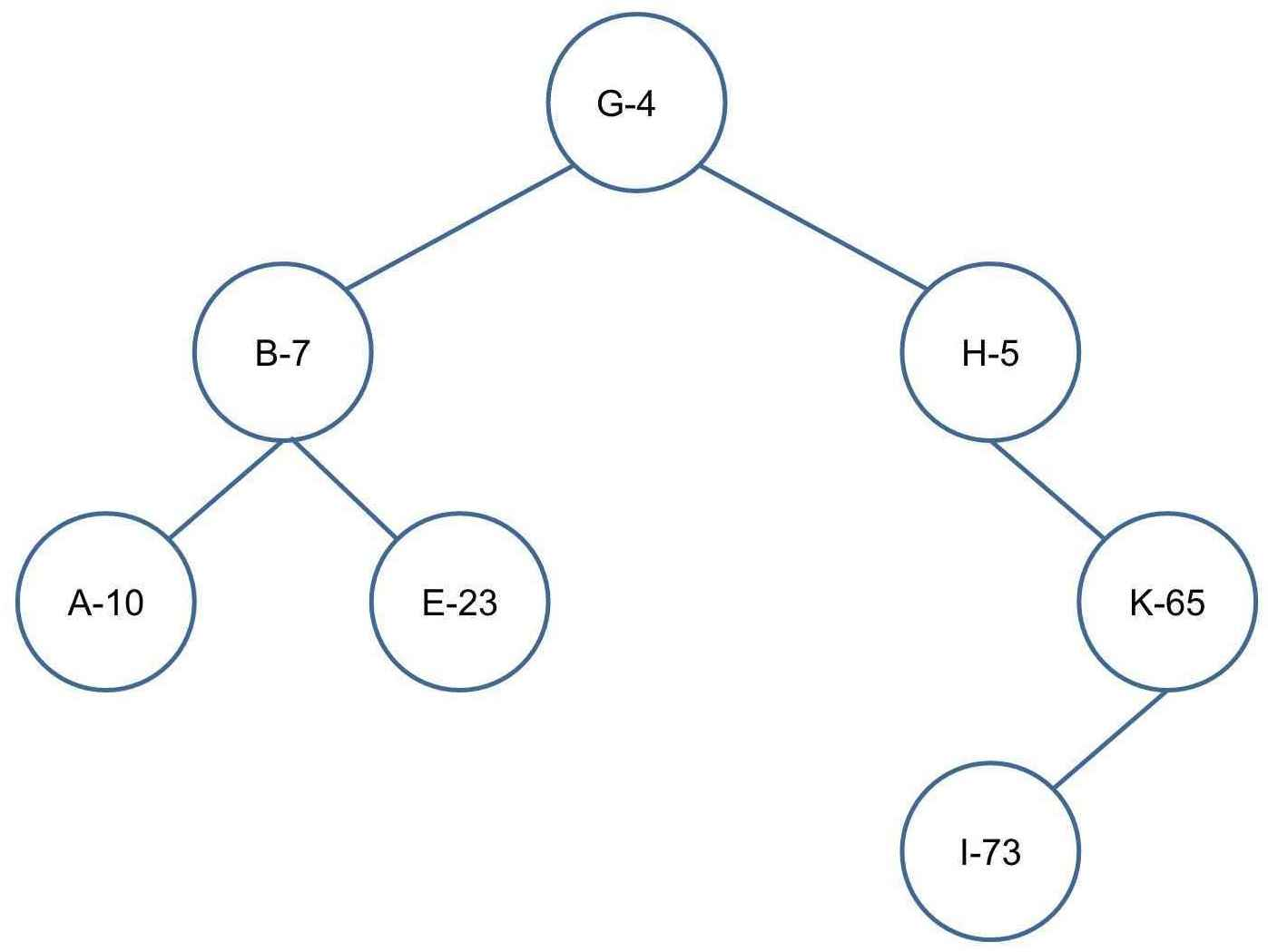插入C-25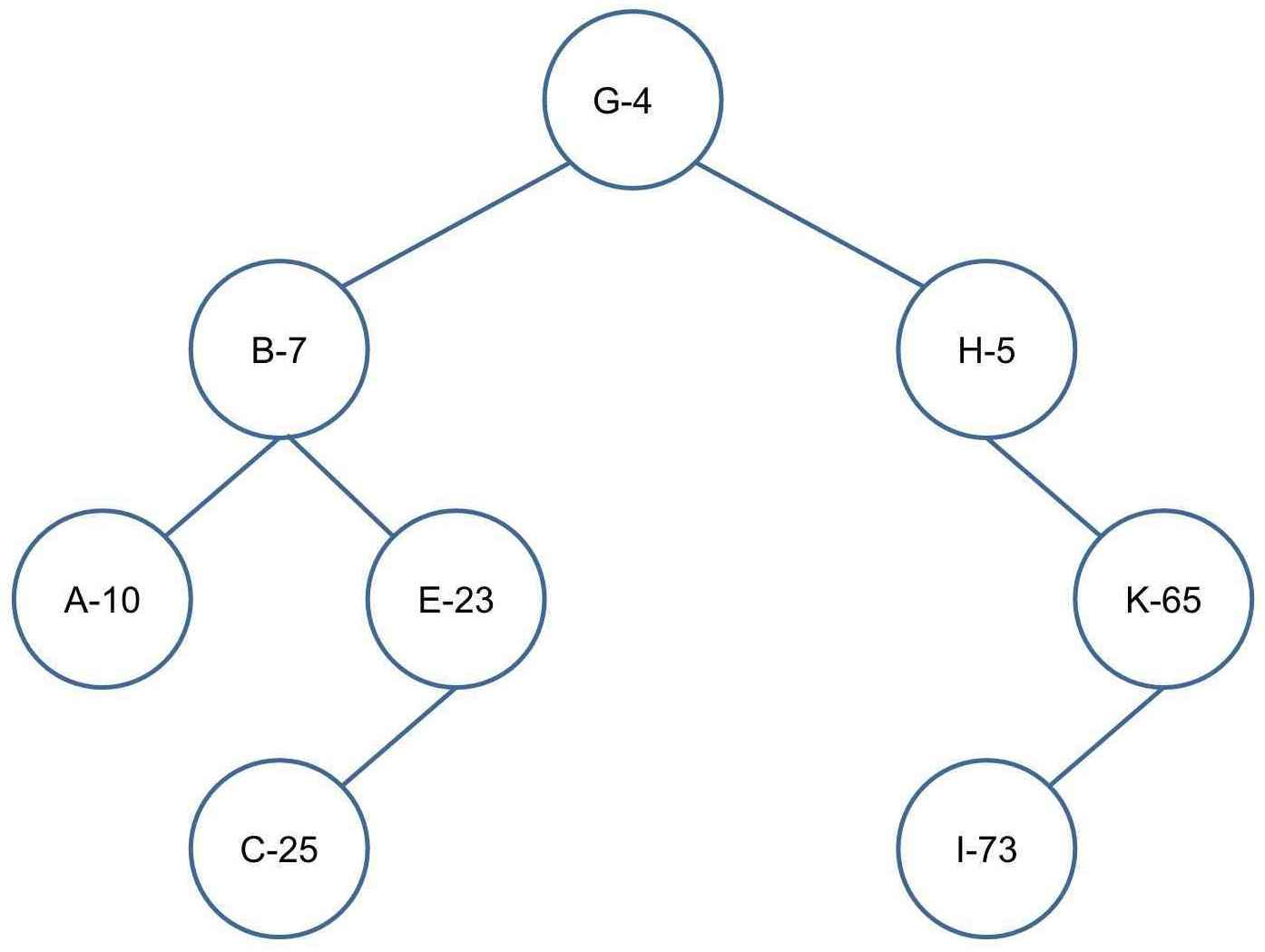插入D-9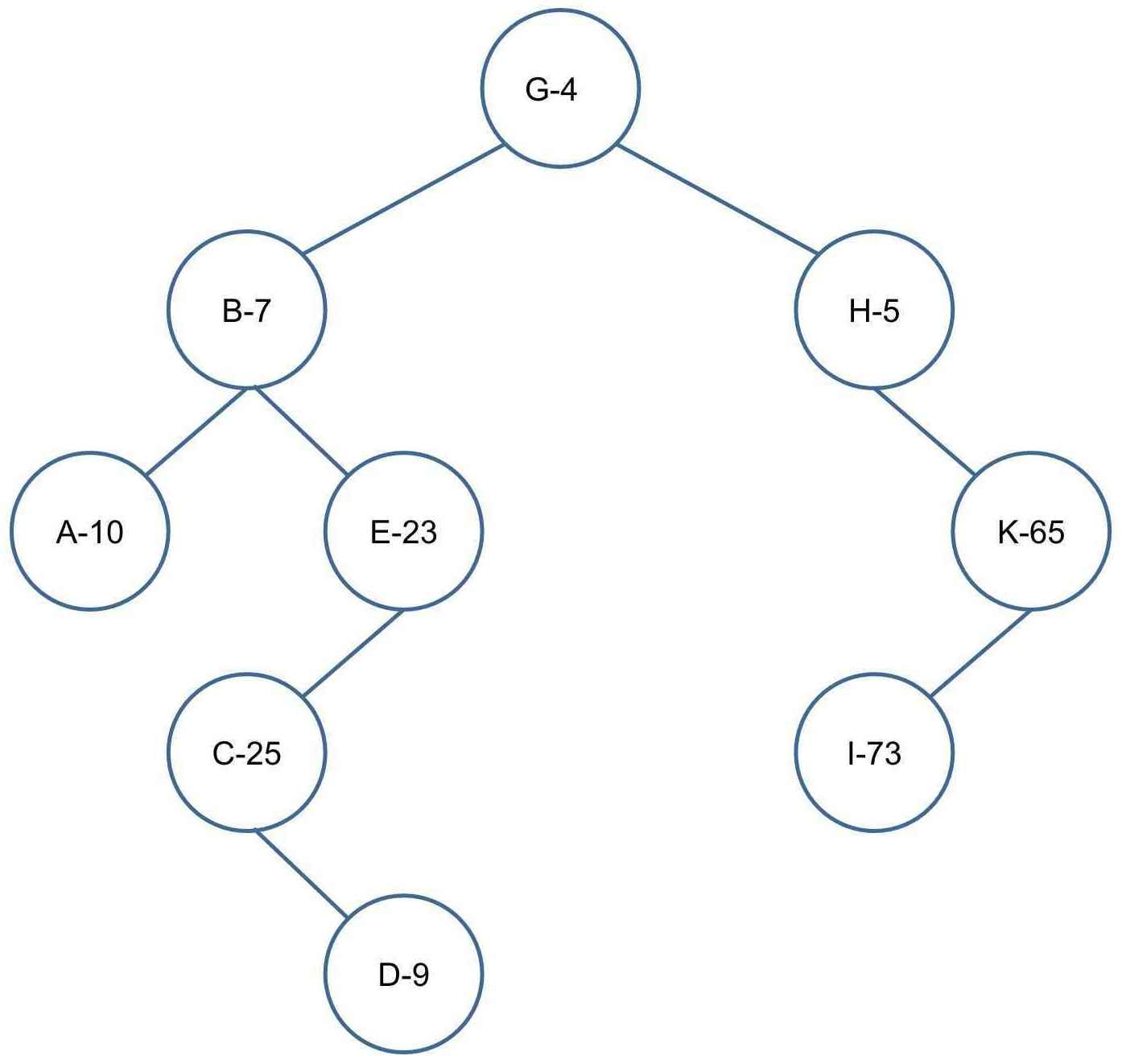左旋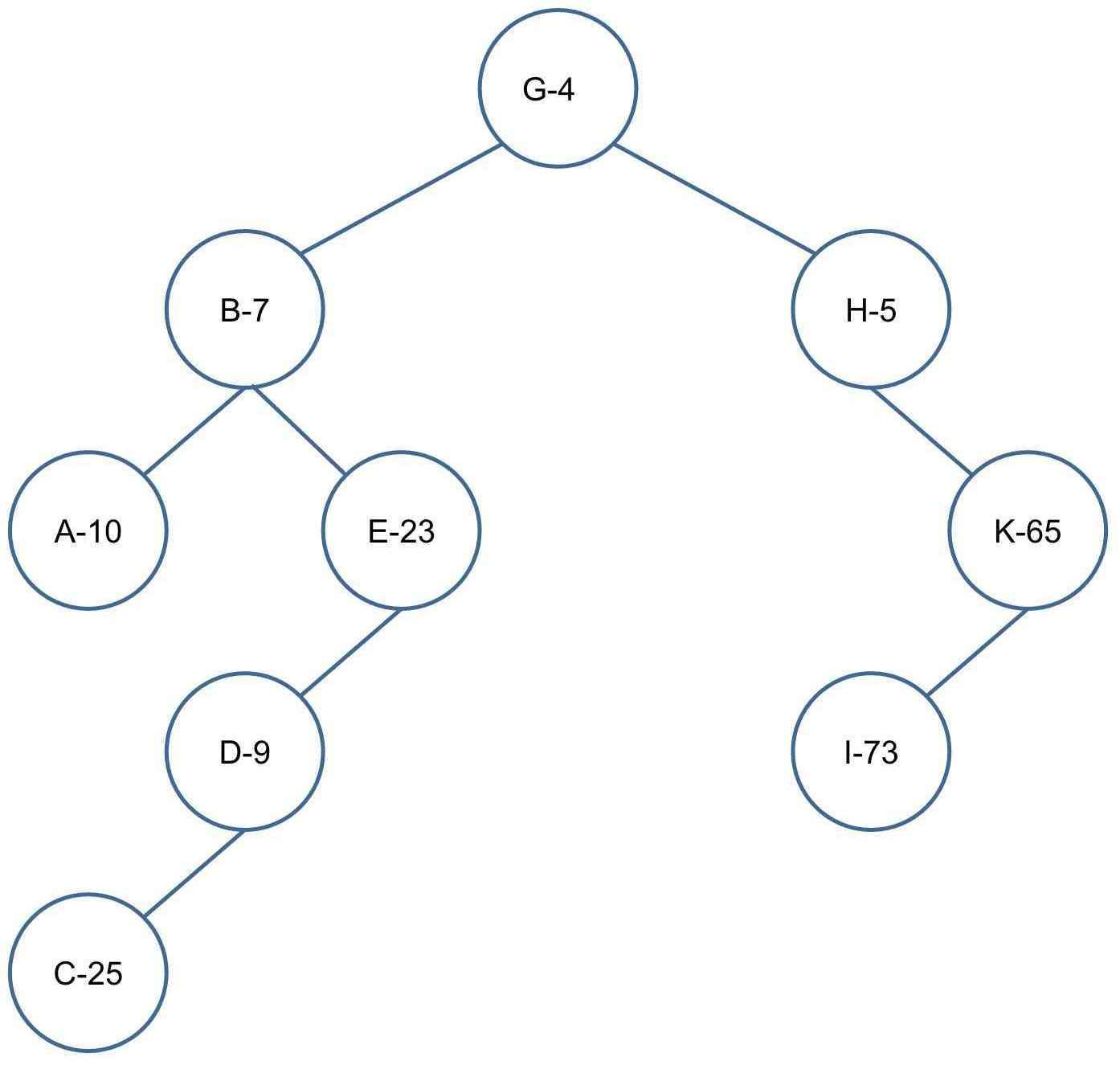右旋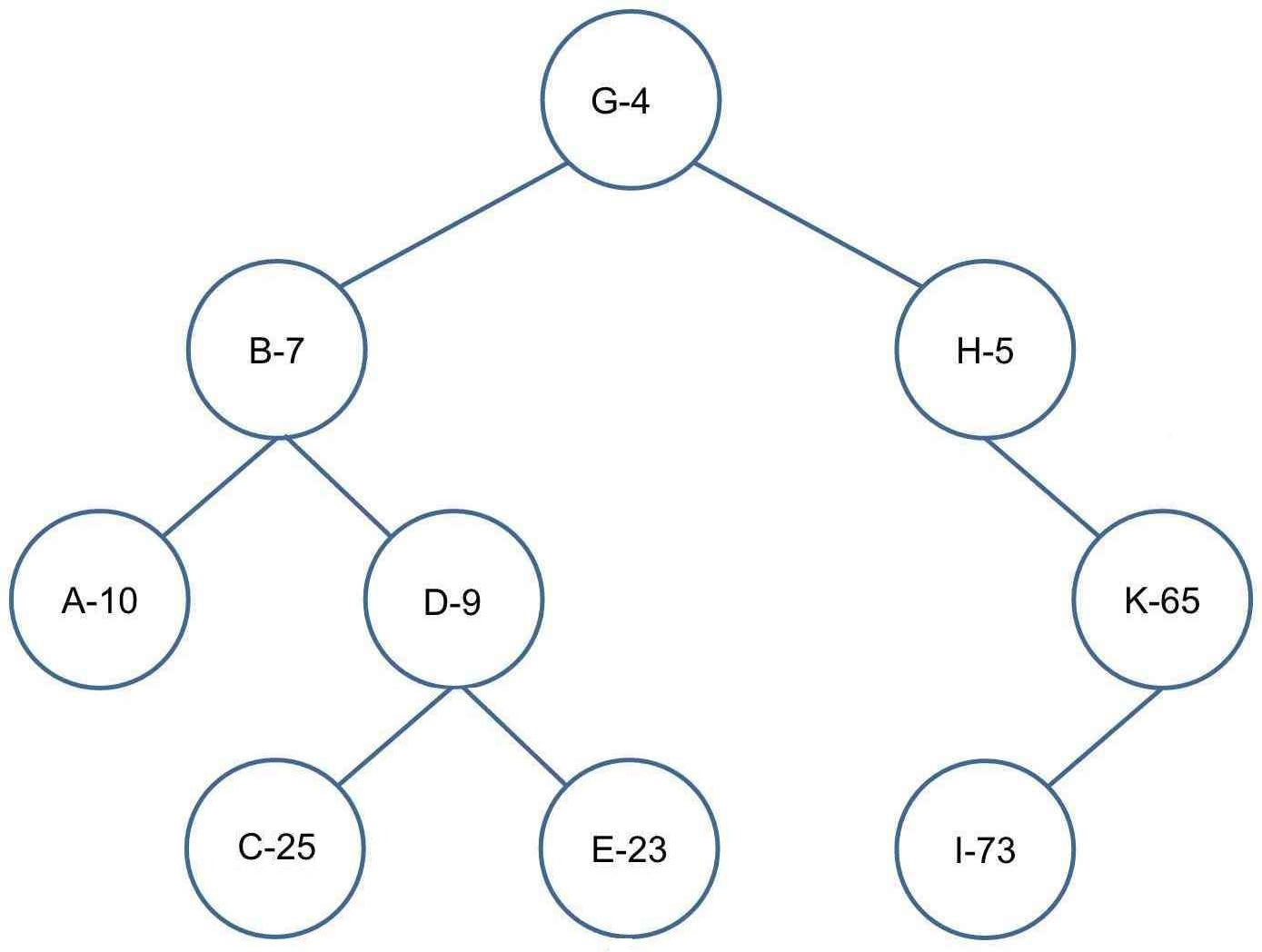插入F-2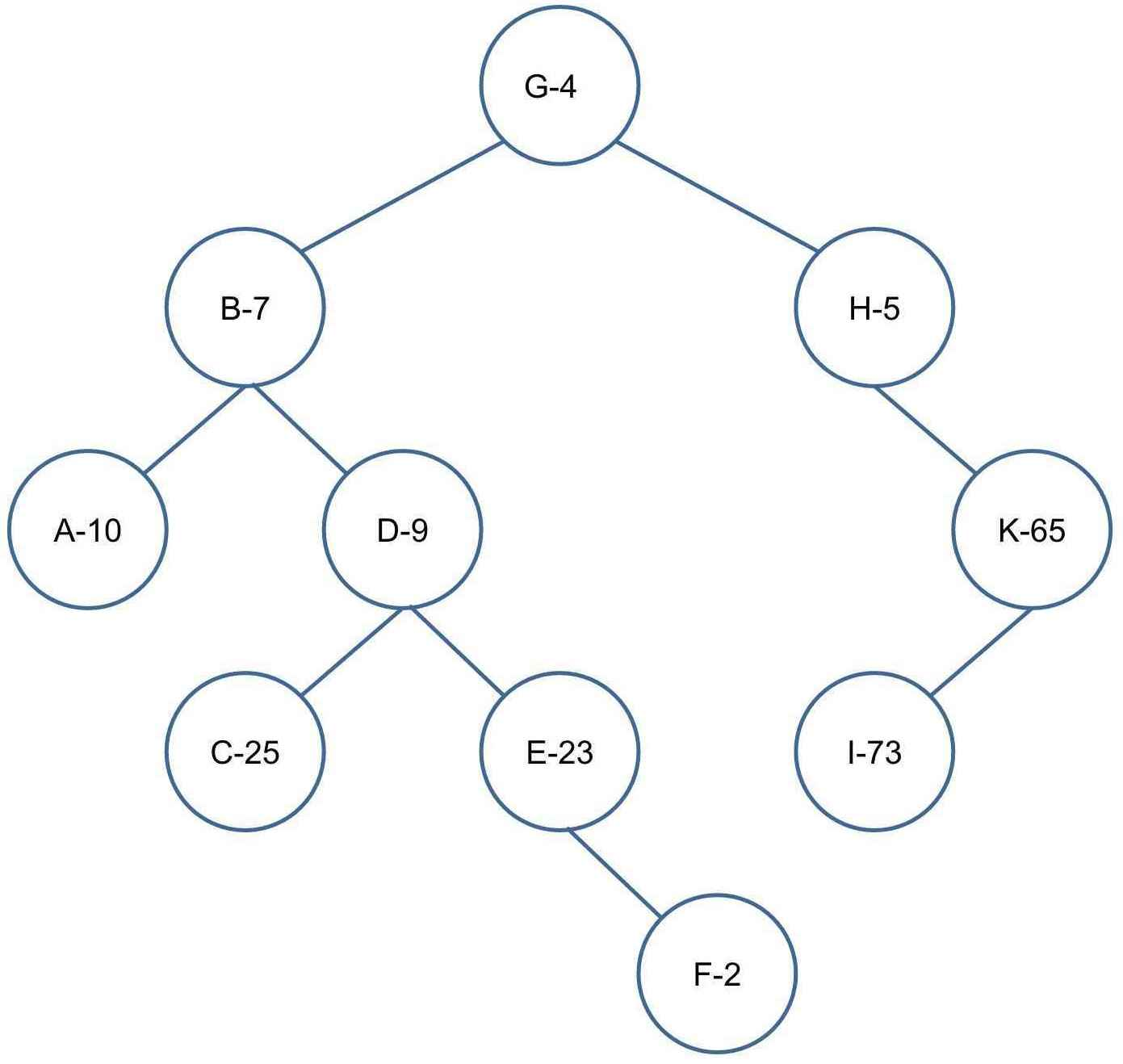左旋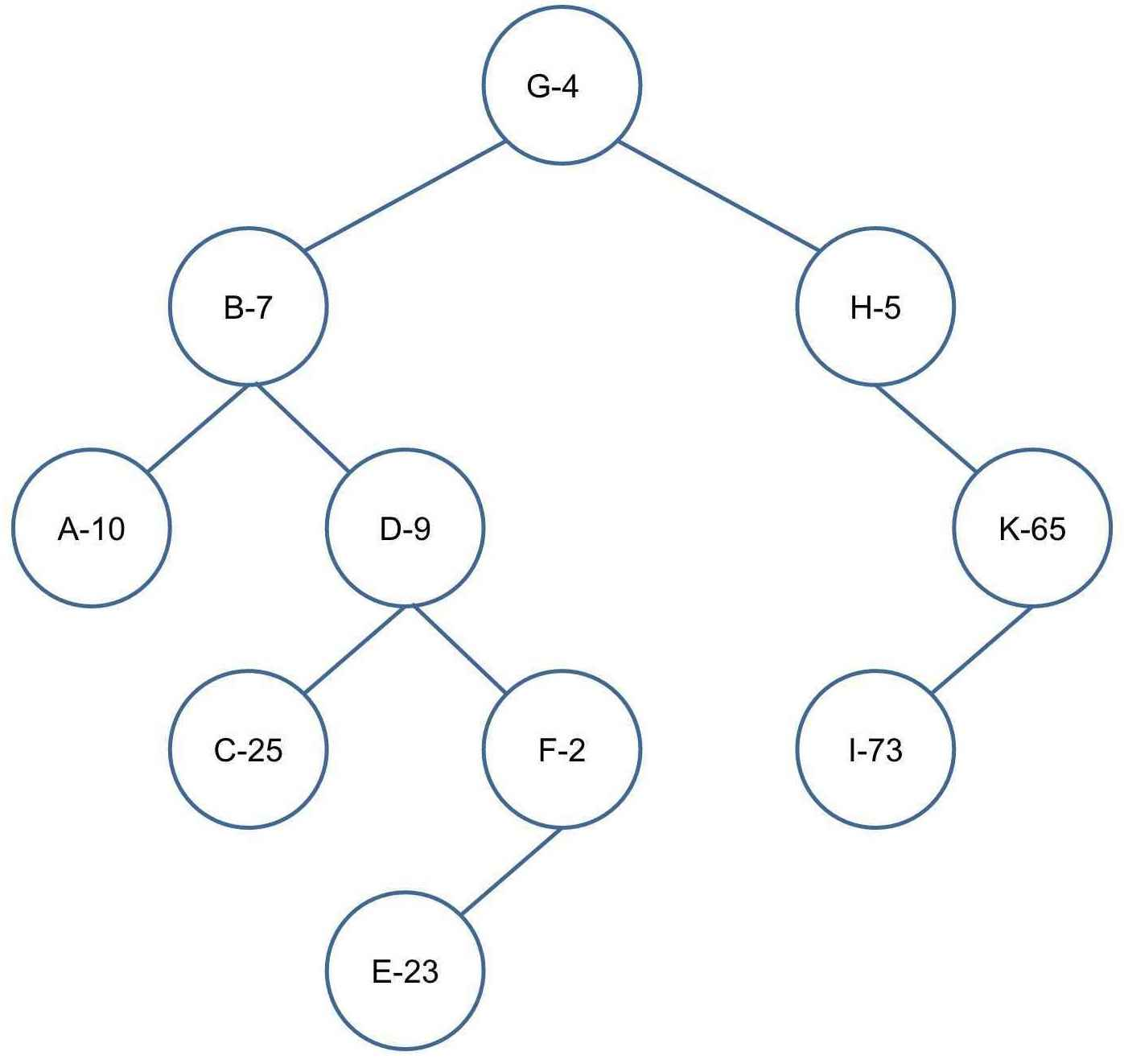左旋左旋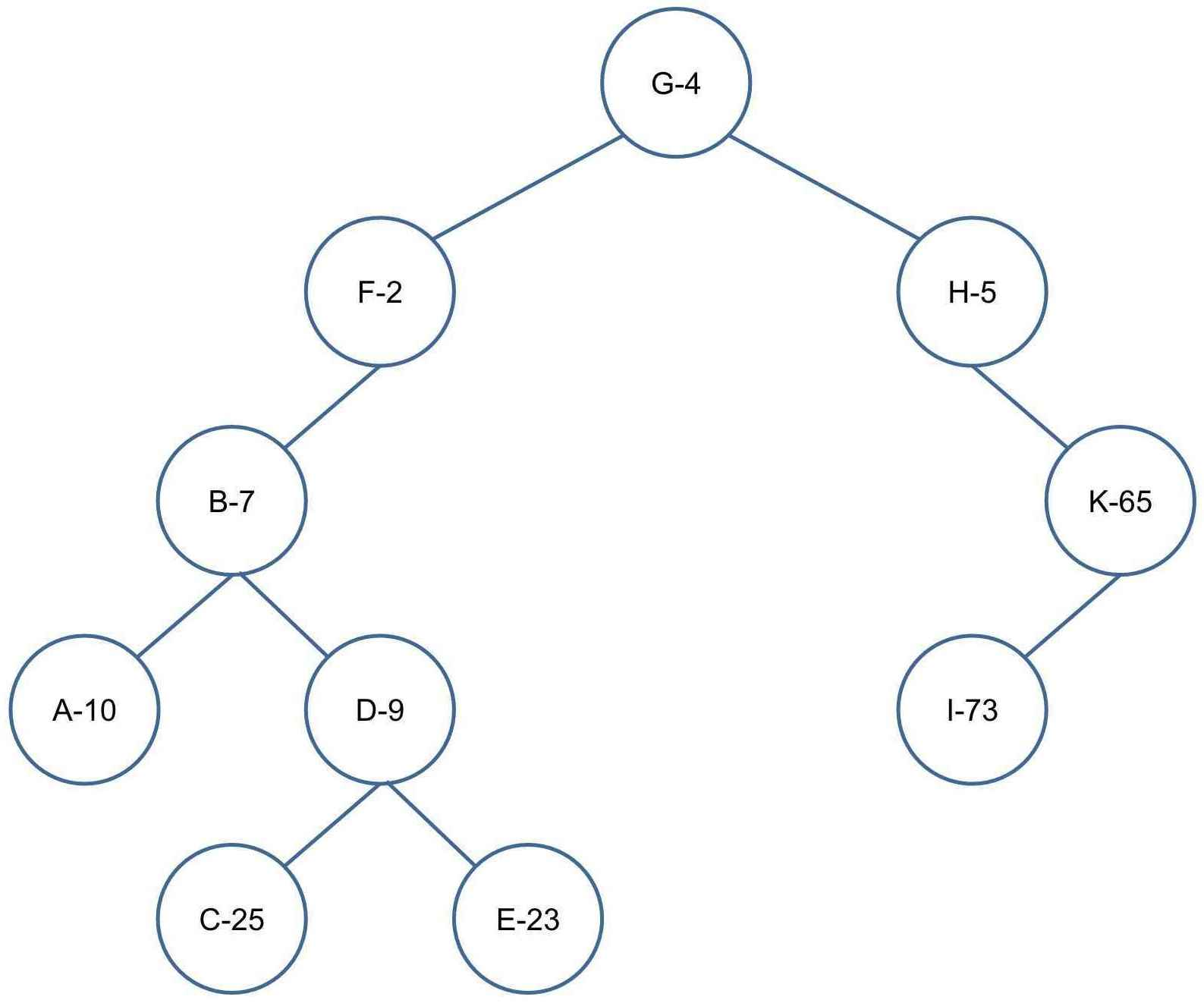右旋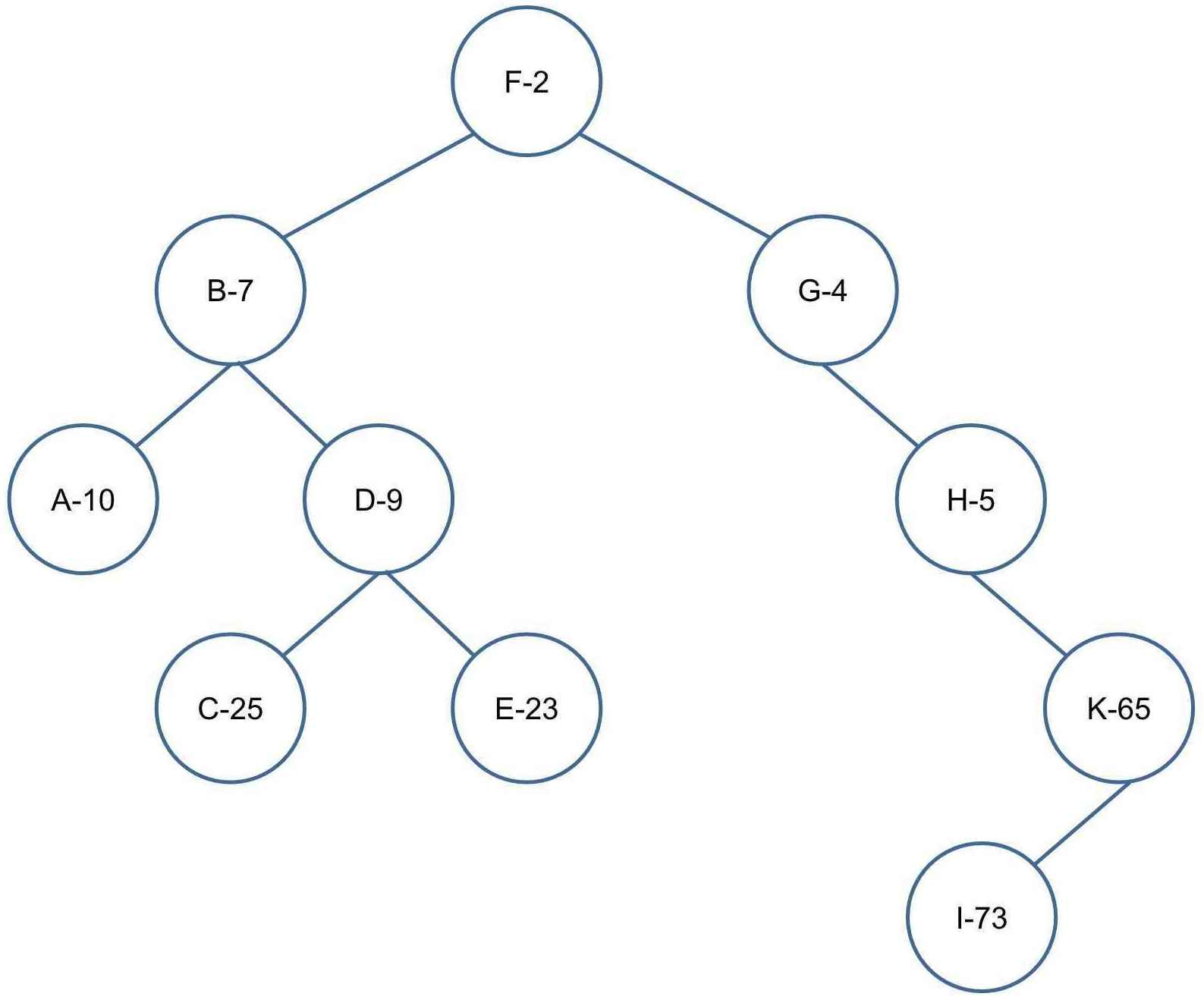2. 删除一个数$k$

利用二叉排序树的性质，先寻找对应的节点，从根节点开始：

(1)$k<o.key$，进入该节点左儿子查找；

(2)$k>o.key$，进入该节点右儿子查找。（注意，在寻找节点的递归过程中，就可以维护路径上节点的$siz$值，即每个经过的节点$siz-1$

找到对应节点时，又分两种情况：

(1)$o.val>1$$o.val-1$即可；

(2)需要删除这个节点，通常采用二叉排序树的删除方法和旋转结合起来，有如下三种情况：

<1>若该节点为叶节点，直接删除即可；

<2>若该节点的子节点中有一个为空，则直接删除该节点，用那个非空的子节点代替；

<3>在其两个子节点中选取优先级较小的节点旋转上来（左儿子——右旋，右儿子——左旋），再向下递归并旋转，直到满足该节点的子节点中至少有一个为空为止。

3. 查询数$k$的排名。

依然利用二叉排序树的性质，排名$k$前的点，中序遍历时就先遍历到；

从根节点开始查找，有如下三种情况：

(1)$k<o.key$，向$o$的左儿子查找；

(2)$k=o.key$，返回$o$的左子树的大小（左儿子的$siz+1$）；

(3)$k>o.key$，给答案加上$o$的左子树的大小以及$o.val$，再向$o$的右儿子查找。

4. 查询排名为$k$的数。

和操作三的思路相似，从根节点开始查找，有如下三种情况：

(1)$k<o$的左子树的大小，向$o$的左儿子查找；

(2)在不满足$1$的情况下，若$k<o$的左子树的大小$+o.val$，即排名为$k$的点不在$o$的左子树上，也不在$o$的右子树上，该节点的关键字就是答案，返回$o.key$

(3)上述两种情况都不满足，即$k$$o$的右子树上，向$o$的右儿子查找，但是向下递归传过去的$k$要减去$o$的左子树的大小和$o.val$（左半边的排名已经计算在内，向右子树查找时需要把它排除在外）；

5. 查询数$k$的前驱（比$k$小的最大的数）。

首先，这个数一定在$k$的左子树上（比$k$小），查找到了$k$的左子树，就要一直向右子树查找（让这个数尽量大），直到查找到空节点为止，第二个过程中不断更新答案$ans$即可；注意$ans$初值赋$-INF$

6. 查询数$k$的后继（比$k$大的最小的数）。

与操作五相反，这里不再过多赘述；

1. 有查询操作时，需要注意是否保证有合法结果，没有合法结果要输出什么；

2. NOI系列比赛中，程序不允许获取当前时间，所以随机数不能撒时间种子，一般以数据范围$n$作为种子；

xqmmcqs

2017-12-04

2021-02-23